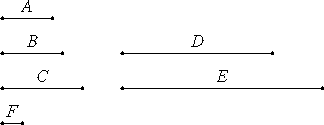# Proposition 17

If a number multiplied by two numbers makes certain numbers, then the numbers so produced have the same ratio as the numbers multiplied.

Let the number A multiplied by the two numbers B and C make D and E.

I say that B is to C as D is to E.VII.Def.15

Since A multiplied by B makes D, therefore B measures D according to the units in A.

VII.Def.20

But the unit F also measures the number A according to the units in it, therefore the unit F measures the number A the same number of times that B measures D. Therefore the unit F is to the number A as B is to D.

For the same reason the unit F is to the number A as C is to E, therefore B is to D as C is to E.

VII.13

Therefore, alternately B is to C as D is to E.

Therefore, if a number multiplied by two numbers makes certain numbers, then the numbers so produced have the same ratio as the numbers multiplied.

Q.E.D.

## Guide

Symbolically, b : c = ab : ac.

This proposition is used very frequently in Books VII through IX starting with the next proposition.